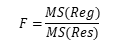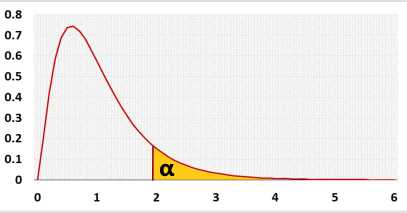# Linear Regression Calculator

## One Variable

Multiple regression with stepwise method and more validations: multiple regression

Constant is zero (Force zero Y-intercept, b0=0)
Header - You may change groups' names to the real names.
Data - When entering data, press Enter or comma, , after each value.
You may leave empty cells, the tool ignores non-numeric cells.

## Information

The tool calculates the Linear equation, R, p-value, outliers and the adjusted Fisher-Pearson coefficient of skewness. After checking the residuals' normality and priori power, the program interprets the results. Then, it draws a histogram, a residuals QQ-plot, a residuals x-plot, and a distribution chart.

Right-tailed F test. Checks if the entire regression model is statistically significant. Why?
Hypotheses
H0: Y = b0
H1: Y = b0+b1X
Test statisticF distribution